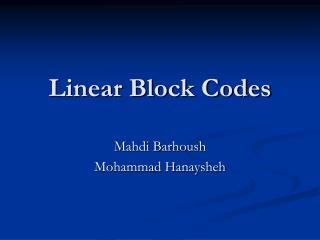Download PresentationLinear Block Codes

# Linear Block Codes - PowerPoint PPT PresentationDownload Presentation## Linear Block Codes

- - - - - - - - - - - - - - - - - - - - - - - - - - - E N D - - - - - - - - - - - - - - - - - - - - - - - - - - -
##### Presentation Transcript

1. Linear Block Codes Mahdi Barhoush Mohammad Hanaysheh

2. Introduction to Block Codes * Block codes introduce controlled amounts of redundancy into a transmitted data stream. * Block code systems divide uncoded data stream into fixed size blocks (k symbol), then add redundancy to each block (n-k symbols) to form the encoded data stream. * (k information symbol )+( n-kparity check symbol) >>> >>>> (n symbol code word) * Parity or redundancy bits are used for error detection and correction.

3. * Here, we have (n, k) code with rate R = k / n. * If the symbol is either 0 or 1 >>> Binary block code, symbols are named bits. * There are 2^n possible code words in a binary block code of length n. * From these, we choose 2^k code words to be mapped to M = 2^k different message. * Thus, a block of k information bits is mapped into a code word of length n selected from the set M = 2 ^ k code words. * Any code has a weight which is the number of nonzero elements that it contains.

4. * Hamming distance is the number of differences between the corresponding elements in any two code words. (measure of difference between any two code words) Ex. dh (1100,1111) = 2 dh (1100,1101) = 1 Then 1100 is closer to 1101 * The smallest hamming distance between any two code words is called the minimum hamming distance dh min. * The idea with error correction codes is to pick the 2^k code words of the 2^n total possible code words which are far enough apart (in terms of Hamming distance) to guarantee you are able to correct a certain number of errors. * dh min = 2*Ct + Dt +1

5. Linearity: * The block code is called linear block code if the addition of any two code words is also a code word. * The addition is performed under Galois Field GF(2) in binary block code. *Linearity implies that the linear block code must contain the all zeros code word.

6. How to generate a linear code ? Using linear algebra and matrices representation: C = m . G Where C is 1 X n code matrix. m is 1 X k message matrix. G is k X n Generation matrix of the code (The rows of the generator matrix are linearly independent and so G has a rank k. )

7. Ex. Let Find the code for m= Solution C = m . G =  . G = 

8. Linear systematic block code: In symmetric form, the code word C is compromised of a k information segment and a set of n-k symbols that are linear combination of certain information symbols determined by the P matrix. m = [m1 m2 m3 m4 ] >>> C = [C1 C2 C3 C4 C5 C6 C7] = [m1 m2 m3 m4 C5 C6 C7] Then, G = [ Ik P] Ikis the identity matrix Pis k X (n - k) matrix

9. Parity check matrix H: The (n, k) linear code can be also specified by an (n –k) X n matrix H Such that any code C satisfies C . T(H) =[000…0]. The above formula implies that G . T(H) = 0 For systematic linear block code H= [ T(P) I n-k ]

10. Example.

11. Types of liner block codes: 1. Hamming code. 2. Cyclic Codes. 3. Polynomial Codes. 4. Reed Solomon Codes. 5. Algebraic Geometric Codes. 6. Reed-Muller Codes. 7. Perfect Codes. 8. Repetition Codes.

12. Hamming code: * (n, k) = ( 2^w -1, 2^w -1- w), w is any positive integer let w =3 >>>> (7, 4) hamming code * In Hamming code, the parity check matrix H ,the n column consists of all possible binary vectors except the all zero vectors. * dh min = 3 >>> can correct one error. * We have 2^k =16 codes such that C . T(H) = 0

13. Hamming code, single error correction: * Suppose that a single error occurred to the transmitted code C In the i th place, the received vector r = C + e(i) e(i) : zero row vector with length n except for the i th position. Using the barity check matrix H for decoding r . T(H) = ( C + e(i) ) . T(H) = C . T(H) + e(i) .T(H) = e(i) . T(H) >>> Gives the location of the error